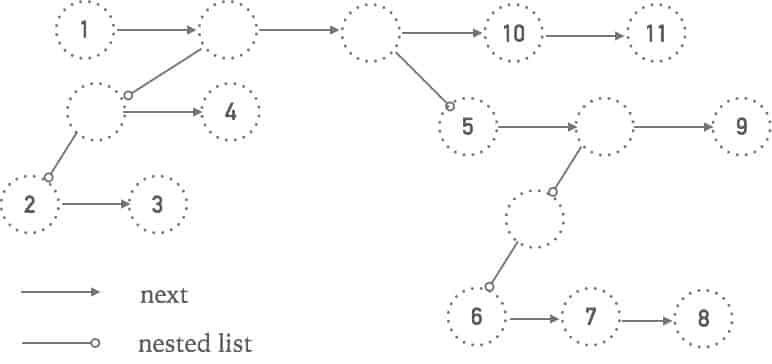Posted by JH

Each node in the list carries a piece of data and a pointer. The data could be in a normal data type such as an integer, or a pointer that points to another list node.

The interviewer gave no specification on how the list node class was defined. You may look at each list node as if it has two pointers - one of them points to the next node; the other points to the ‘data’ which could be either a nested list or an actual value. In the node class will have a function that tells whether the node carries a value or a nested list.# Solution

To flatten the nested list, every time a node N carrying a nested list is found, run flattenNestedList(N) recursively on node N. When the nested list within N is flattened to a 1 dimensional list L, simply append N.next to the tail of L and append L to N’s previous node.
Note that this is a single linked list. Therefore when iterate to N, keep track of the previous node to N as well.

Variant questions such as 'Flatten Nested List' and 'Flatten nested Iterator' can be solved by similar approach with recursion.

# Implementation

``````class Wrapper {
ListNode tail;
public Wrapper(ListNode h, ListNode t) {
tail = t;
}
}

ListNode dummy = new ListNode(0);
ListNode node = dummy;
while(node.next != null) {
if(node.next.data != null) {
node = node.next;
} else {
Wrapper flattened = flatten(node.next.list);
if(flattened.tail != null) {
flattened.tail.next = node.next.next; //delete the node with nested list
node = flattened.tail;
} else {
node.next = node.next.next;
}
}
}
return new Wrapper(dummy.next, node);
}
``````